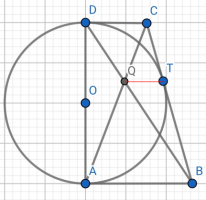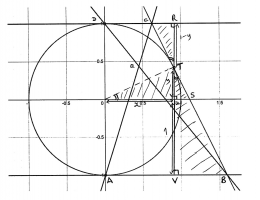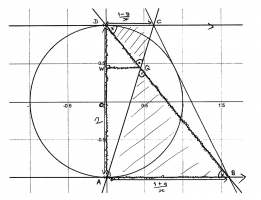# Proof

#### topsquark

##### Senior Member
Since ABCD is a right trapezoid maybe it would be easier to show that TQ is parallel to AB and CD, which are themselves perpendicular to AD.

-Dan

•Dr.Peterson

#### Dr.Peterson

##### Elite Member
How are CD and CT related?

How are QD and QB related?

•topsquark

#### canvas

##### New member
AC and BD are diagonals in the right trapezoid ABCD.
Q - the point of intersection of diagonals.

#### pka

##### Elite Member
Can you show that [imath]\overleftrightarrow {AB}||\overleftrightarrow {QT}\text{ or }\overleftrightarrow {DC}||\overleftrightarrow {QT}~?[/imath]

#### canvas

##### New member
I see that it's equivalent with our argument but I don't have any idea how to prove it, any hints?

#### JeffM

##### Elite Member
You seem to be giving us the problem in pieces.

But consider $\triangle DCQ$ and $\triangle AQB$.

#### canvas

##### New member
I see that these triangles are similar but I can't find any connection with TQ and these triangles.

#### topsquark

##### Senior Member
You seem to be giving us the problem in pieces.

But consider $\triangle DCQ$ and $\triangle AQB$.
You mean [imath]\triangle[/imath]DQC and [imath]\triangle[/imath]BQA...

-Dan

#### Dr.Peterson

##### Elite Member
How are CD and CT related?

How are QD and QB related?
Please answer these questions specifically. We need to see you actually thinking, in order to direct your thoughts. If some triangles are similar, which ones, and why? (It helps if you use the reply button so we can tell what question you are answering.)

My first question relates to an important fact about tangents. The second has to do with similar triangles.

#### canvas

##### New member
Yes, these triangles are similar by angle-angle-angle, DC=CT and TB=AB but I can't find any connection with TQ.

#### Dr.Peterson

##### Elite Member
Yes, these triangles are similar by angle-angle-angle, DC=CT and TB=AB but I can't find any connection with TQ.
Try thinking about triangle BQT (or CQT). Do you see that if the conclusion is true, then since TQ is parallel to AB and to CD, therefore BQT is similar to BDC? You'd like to prove that it is, starting with what you know.

Either of these approaches is easier to see if you draw some additional line segments, such as the TQ you asked about. Doing so reveals additional triangles to consider.

#### pka

##### Elite Member
If you know that [imath]\overleftrightarrow {AB}||\overleftrightarrow {QT}[/imath]
then because [imath]\overleftrightarrow {AB} \bot \overleftrightarrow {AD}[/imath] (WHY?)

#### canvas

##### New member
AB is perpendicular to AD because ABCD is right trapezoid, but I don't how to prove that TQ is parallel to AB or CD. I will just skip that problem, I've wasted too much time on that proof. Thank you guys

#### canvas

##### New member
I don't *know* how

#### pka

##### Elite Member
Can you find alternate interior angles that are congruent?
What can you show about [imath]\angle DCQ~\&~\angle CQT~?[/imath]

#### Dr.Peterson

##### Elite Member
AB is perpendicular to AD because ABCD is right trapezoid, but I don't how to prove that TQ is parallel to AB or CD. I will just skip that problem, I've wasted too much time on that proof. Thank you guysWhat is the ratio DQ : QB?

What is the ratio CT : TB? (In fact, what are CT and TB?)

Can you use those to show that QTB is similar to DCB?

#### lex

##### Full Member
It may be more straightforward to deal with the equations of the lines rather than similar triangles.
Taking AD to be vertical, all we have to show is that Q has the same Y co-ordinate as T.

Take the radius of the circle to be 1 (wlog), O (0,0) and T has co-ordinates (x,y)
(Note [imath]x^2+y^2=1[/imath])

Then OT has gradient [imath]\tfrac{y}{x}[/imath] and therefore CB has gradient [imath]-\tfrac{x}{y}[/imath] (assuming, that CB is a tangent at T and therefore perpendicular to OT).

[imath]\therefore[/imath] CB goes through [imath](x,y)[/imath] with gradient [imath]-\tfrac{x}{y}[/imath]

Eqn of CB: [imath]\boxed{\;\boldsymbol{Y}-y=-\tfrac{x}{y}(\boldsymbol{X}-x)\;}[/imath] (*)

D(0,1) A(0,-1)

C is on CB (*) and has Y co-ordinate = 1 [imath]\hspace4ex \therefore \boldsymbol{X}=\tfrac{y(1-y)}{-x}+x[/imath]
[imath]\hspace41ex \boldsymbol{X}=\tfrac{1-y}{x} \hspace6ex[/imath] (since [imath]x^2+y^2=1[/imath])
C[imath]\left(\tfrac{1-y}{x},1\right)[/imath]

Similarly B is on CB (*) and has Y co-ordinate = -1[imath]\hspace4ex \rightarrow \boldsymbol{X}=\tfrac{1+y}{x}[/imath]

B [imath]\left(\tfrac{1-y}{x},-1\right)[/imath]

Now equation of AC: A(0,-1) C[imath]\left(\tfrac{1-y}{x},1\right)[/imath]
[imath]\boxed{\,AC\,} \hspace2ex \boldsymbol{Y}=\tfrac{2x}{1-y}(\boldsymbol{X})-1[/imath]

Eqn of DB: D(0,1) B[imath]\left(\tfrac{1+y}{x},-1\right)[/imath]
[imath]\boxed{\,DB\,} \hspace2ex \boldsymbol{Y}=-\tfrac{2x}{1+y}(\boldsymbol{X})+1[/imath]

Q is at the intersection of [imath]\boxed{\,AC\,}, \boxed{\,DB\,}[/imath]
[imath]\rightarrow \left(\tfrac{2x}{1-y}+\tfrac{2x}{1+y}\right)\boldsymbol{X}=2[/imath]
[imath]\tfrac{4x}{1-y^2} \boldsymbol{X}=2[/imath]
[imath]\boldsymbol{X}=\tfrac{x}{2} \hspace10ex[/imath] note [imath]1-y^2=x^2[/imath]
[imath]\therefore \boldsymbol{Y}=y \hspace8ex[/imath] using e.g. DB, note [imath]1-x^2=y^2[/imath]

Q.E.D

If you do want to do this using similar triangles:Assuming CB is tangent to the circle at T, then:
[imath]\bigtriangleup OTU \backsim \bigtriangleup TSU \backsim \bigtriangleup TBV[/imath]
[imath]\hspace10ex \bigtriangleup TSU \backsim \bigtriangleup TCR \hspace5ex (\backsim\;[/imath] similar[imath])[/imath]

[imath]TU=y, US=\tfrac{y}{x} . y = \tfrac{y^2}{x}, VB=\tfrac{1+y}{y} . \tfrac{y^2}{x}=\tfrac{y+y^2}{x}\\ \hspace15ex US=\tfrac{y^2}{x}, RC=\tfrac{1-y}{y} . \tfrac{y^2}{x} = \tfrac{y-y^2}{x}[/imath]

[imath]DC = DR - RC[/imath]
[imath]=x-\tfrac{y-y^2}{x}\\ \boxed{\;DC=\tfrac{1-y}{x}\;} \hspace5ex[/imath] (since [imath]x^2+y^2=1[/imath])

[imath]AB = AV + VB = x+ \tfrac{y+y^2}{x}\\ \boxed{\;AB= \tfrac{1+y}{x}\;}[/imath]Assuming CD and AB are tangents at D and A respectively, then CD and AB are parallel, therefore [imath]\bigtriangleup DCQ \backsim \bigtriangleup BAQ[/imath]

Now [imath]AB =\tfrac{1+y}{1-y} DC\\ \therefore QB=\tfrac{1+y}{1-y} DQ\\ \therefore DB = DQ + \tfrac{1+y}{1-y} DQ\\ DB = \tfrac{2}{1-y} DQ[/imath]

Now [imath]\bigtriangleup DWQ \backsim \bigtriangleup DAB, \hspace3ex \text{ and from previous line s.f. } \tfrac{1-y}{2}[/imath] (to get sides in [imath]\bigtriangleup DWQ[/imath])

[imath]\therefore WQ = \tfrac{1-y}{2} . AB = \tfrac{1-y}{2} . \tfrac{1+y}{x}=\tfrac{1-y^2}{2x}=\tfrac{x^2}{2x}=\tfrac{x}{2}[/imath]

[imath]DW = \tfrac{1-y}{2} DA = \tfrac{1-y}{2} . 2 = 1-y[/imath]
i.e. [imath]WO=y[/imath]

Now [imath]\hspace1ex QO=WO[/imath]
[imath]\therefore QO=y[/imath]
[imath]\therefore[/imath] Q is at the same height as T.
Q.E.D.

•Subhotosh Khan